﻿ Technology-Based Startup Valuation Using Real Options with Edgeworth Expansion

Technology-Based Startup Valuation Using Real Options with Edgeworth Expansion

Gastón Milanesi, Gabriela Pesce, Emilio El AlabiOPEN ACCESSPEER-REVIEWED

Technology-Based Startup Valuation Using Real Options with Edgeworth Expansion

Gastón Milanesi1,, Gabriela Pesce1, Emilio El Alabi1

Abstract

There exists extreme difficulties while trying to valuate, using traditional valuation methods, startup firms which are dedicated to technological development. Among those methods, we could mention the balance sheet-based ones, the relative valuation ones, the cash flow discounting-based ones, and the goodwill-based ones. Those difficulties are the absence of comparable companies, the inexistence of historical data, the complexity to estimate volatility, and the number of intangible assets which give worth to the firm. This paper proposes to valuate this type of entrepreneurships using real options theory making adjustments that allow us to abandon the assumption of normal returns. Methodologically, we use real options theory adapted through Edgeworth expansion. It allows abandoning the probability of normal distribution assumption incorporating higher moments such us asymmetry and kurtosis. Obtained results let us show how the firm’s value and its strategic options are affected by stochastic higher moments’ behavior. These are often not considered because of assuming a normal behavior related to a random path of the underlying assets.

At a glance: Figures

1
Prev Next

• Milanesi, Gastón, Gabriela Pesce, and Emilio El Alabi. "Technology-Based Startup Valuation Using Real Options with Edgeworth Expansion." Journal of Finance and Accounting 1.2 (2013): 54-61.
• Milanesi, G. , Pesce, G. , & Alabi, E. E. (2013). Technology-Based Startup Valuation Using Real Options with Edgeworth Expansion. Journal of Finance and Accounting, 1(2), 54-61.
• Milanesi, Gastón, Gabriela Pesce, and Emilio El Alabi. "Technology-Based Startup Valuation Using Real Options with Edgeworth Expansion." Journal of Finance and Accounting 1, no. 2 (2013): 54-61.

 Import into BibTeX Import into EndNote Import into RefMan Import into RefWorks

1. Introduction

Over the last few years, valuation models have gone from being a bunch of complex methods to an essential tool to valuate financial and real assets . In real assets, real options theory helps us valuing the strategic flexibility contained in investment decisions, and calculating companies’ worth . Its accuracy stands on the existence of perfect, efficient, and complete markets. Through them, we will be able to replicate risk involved in that portfolio, and to assume Gaussian stochastic behavior in random variables. However, these conditions are weaknesses in certain real assets . Specifically, these drawbacks emerge in young companies or startups, and boost when they develop technologies.

While trying to valuate technology-based young companies, traditional valuating methods such as the accounting ones, the based on multiples ones, the cash flow discounting ones, and the mixed ones present numerous limitations generated by:

Having a great number of intangible assets provokes that static values that come up from accounting methods undervalue the organization.

The absent of comparable companies complicates the application of valuation multiples methods or the practice of industrial average to estimate discount rates on systematic risk.

The inexistence of historical data generates barriers to find an adequate discount rate, a return rate, and a growth rate from a theoretical point of view.

The difficulty of estimating volatility because of the great uncertainty associated to technology-based startup firms.

In order to value these types of companies, we need to apply real options theory to capture and incorporate the entrepreneurship’ strategic flexibility like the possibility of expansion or transferring licenses, rights, and/or patents. Nonetheless, this is not the integral solution since valuating startup firms with classic models, Black and Scholes in continuous time and binomial method in discrete time, present the following difficulties: (a) In general, there are not any financial assets in the market that allow us to replicate neither project risks nor comparable real assets because of the importance of human capital; (b) involved stochastic processes could deviate themselves from the classical paradigm of normal probability distribution. Thus, we come across the necessity of finding tools that model this particular real assets situation.

Motivated on the indicated limitations, this paper proposes a model based on the classical binomial valuation method [3, 8, 14]. It incorporates stochastic higher moments such as asymmetry and kurtosis through inconstant certain equivalent coefficient to project future scenarios where the underlying asset stochastic process (project value) gets away from normal behavior [10, 11]. Our proposed model objective is to incorporate possible values that come up from sensitizing asymmetry and kurtosis on the stochastic process. We should not miss the binomial model’s simplicity and intuition to value real options. Next, we will summarize the main pillars where the proposed model is built:

Subjective probabilities . It is a salient characteristic in technology-based firms (TBF) because of the company’s innovative level, its important participation of intellectual capital, and its inexistence of a similar financial asset on capital markets. As a consequence, we frequently do not have observations or frequencies related to stochastic movements of a benefit function(1). This makes impossible the use of objective probabilities or observations.

The use of Marketed Asset Disclaimer (MAD) approach  to determine project risk and other parameters of the binomial process because of the inexistence of comparable financial assets.

The addition of higher moments altering the binomial function, estimating implied probabilities and applying certain equivalent coefficients that allow us to incorporate asymmetry and kurtosis.

This paper has the following structure: first, we expose alterations in the binomial function theoretically. Moreover, we describe value projection incorporating higher moments, and implied probabilities’ estimation. Afterwards, we depict our model functioning applying a TBF’s valuation with an option to sell and/or selling a license. Through it, we sensitize different values for higher moments, and present strategic decisions’ impact in value.

2. Literature Review

2.1. Startups and Technology-Based Firms (TBF)

Organizations’ lives evolve through a series of identifiable stages and with particular characteristics that, as a whole, construct a life cycle. Each of these phases has a relationship with firm’s size and age. This research focuses exclusively on young companies that are usually characterized by high potential returns but with a great level of uncertainty about the proposed cash flow. The first cycle stage is critical. It is known as startup or valley of death (this is because of firms’ high mortality rate presented in this phase). It goes from the start of their activities until the moment when they pass through the break-even point, stabilize their sales, and confirm that they are durable businesses. This period’s length depends on the kind of entrepreneurship and its economic sector, being longer on TBF .

Techonology-based firms (TBF) are characterized by developing new technologies based on knowledge, and their value comes mainly from intangible assets such as know-how. They are innovative and dynamic entrepreneurships which have great potential to generate aggregated value and growth.

In order to incorporate strategic flexibility on these young projects based on technological development, we have used real options [1, 2, 7]. We have found that this theory allows us having flexibility in these types of firms, but having strong assumptions on probability distributions of returns.

2.2. Option Pricing with the Edgeworth Expansion

Edgeworth transformation allows us to estimate corrected probabilities. This enables us to calculate the underlying asset’s projected value and, also, the variable certain equivalent coefficients used through the estimation recursive process in real options.

Through the stochastic process to project the underlying value (project value), we use the binomial probability function, b(x). Being n+1 final nodes and j=0, 1, 2,…n are positions that the variable occupies on each node. The number of possible paths is obtained through the following expression: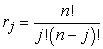(1)

On each position, the random variable’s value x is represented through equation 2, and the binomial probability function b(x) for each node is represented through equation 3.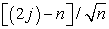(2)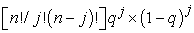(3)

Equation 3 determines binomial probabilities for nodes resulting from the underlying projection. Binomial method to valuate options supposes asymmetry and kurtosis’ inexistence, higher moments. However, several phenomenons do not present distributions that adjust themselves to normality laws. Therefore, it is necessary to incorporate stochastic higher moments to approach the best entrepreneurship value.

In order to incorporate asymmetry (E) and kurtosis (K) to the stochastic process in the binomial method, we need to transform function b(x) (Equation. 3). This is possible through a new function, f(x), known as “Edgeworth density function” . On the binomial function b(x), values related to four moments (mean, variance, asymmetry, and kurtosis) are: E(x) = 0; E(x2) = 1; E(x3) = 0; E(x4) = 3. Supposing a different value from 0 and 3 to higher moments involves stop assuming normality, and requires applying Edgeworth transformation on the original function. Its result is a new function f(x) where the following moments are captured: E(x) = 0; E(x2) = 1; E(x3) = Ε, E (x4)=K.

Therefore, we firstly must calculate the transformation function W(x) using the following expression(2):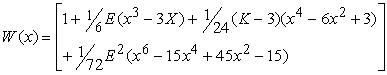(4)

Transformed function is the product of equations 3 and 4 on each node f(x) = b(x).W(x). The expansion is only an approach being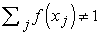. We must scale probabilities so they sum up 1. Thus, we must replace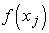with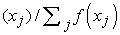.

Secondly, once we have obtained the adjusted density function, we proceed to estimate mean (M) and its variance (v2);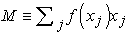(5)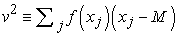(6)

Using equations 5 and 6, we come up with the necessary parameters to standardize the random variable (projected value or intrinsic value).

Thirdly, stochastic higher moments are incorporated in mean and variance. Transformation function W(x) is applied over the binomial function b(x), making the transformed function f(x). At the same time, random variables xj are replaced by new standardized ones through equation 7’s expression.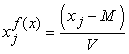(7)

Having a new function f(x) and incorporating higher moments to mean and variance, we proceed to project the underlying asset’s value.

Project value, which is the random variable on each node, is noted as Vj. Thus, we use equation 8 to project the underlying asset’s value on each node employing the corrected function f(x). Its supplies are a growth rate (μ), probabilities obtained from the corrected function f(x) noted as Pj=f(xj), and standard deviation σ.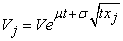(8)

Previous to its estimation, we need to operate over equation 9 in order to obtain the growth rate (μ).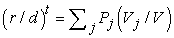(9)

Variables involved in the equation are: V= project’s intrinsic value at initial moment; r= risk free factor equivalent to (1+rf); d= return factor generated by the asset; t= time until deadline; μ= expected risk neutral increment from logarithm Vj/V, and σ= logarithm volatility of Vj/V.

Once we substituted equation 8 on equation 9, applied logarithms, and cleared towards a risk neutral increment (μ), we obtain equations 10 and 11.(10)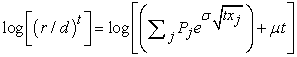(11)

We get the following equation to develop the increasing rate incorporating higher moments, a necessary supply for equation 8.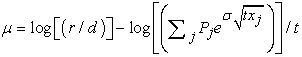(12)

Conceptually, variation rate μ is similar to the one used to estimate risk neutral values assuming a lognormal distribution in prices; μ=[log(r/d)-1/2σ2] . While incorporating higher moments, we consequently abandon a lognormal distribution assumption. Using these elements, we are set to project values for different nodes. Equation 13 arrives to the present value that matches the projected values’ succession.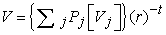(13)

On this case, Vj is estimated from equation 8, Pj=f(xj) by equations 4 and 7 and r which is the risk-free discount factor.

Implied probabilities that are going to be used on the implied binomial lattices are solved through Rubinstein recursive procedure :

Step 1: Process begins on the last nodes of the lattice estimating probabilities using the following expression (Eq. 14).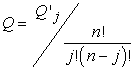(14)

Defining S as the underlying asset, the two adjacent nodes are (Qt+ St+; Ot- St-). These probabilities are conditioned by the precedent node (Qt-1; St-1).

Step 2: The branch’s probability associated to node (Qt-1) is equal to the sum of the posterior adjacent nodes’ probabilities: . The precedent node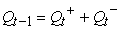summarizes movements and probabilities of the underlying asset (Qt+ St+; Qt- St-).

Step 3: On each node, we obtain certain equivalent coefficients applying conditional probability (eq. 15 and 16).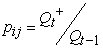(15)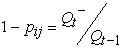(16)

Step 4: St-1 comes out of the product between posterior adjacent branches’ values (St+ ; St-) and certain equivalent coefficients (pjt; 1-pjt). We apply a risk-free rate corresponding to the time interval.

Step 5: We apply recursive method to value the underlying asset (Eq. 17) and the option (Eq. 18).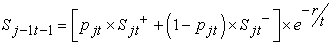(17)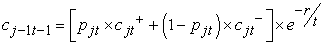(18)

3. Valuation Using Real Option Incorporating Higher Moments: Analysis of a Case

We will use a R&D project on a new technology as an example to portray real options’ behavior, binomial lattices, and higher moments’ integration.

3.1. Case Details: Technology Base Startup Enterprise

The firm is integrated by a group of college researchers and private risk capital. The entrepreneurship’ objective is to create monitoring and radar systems for blind spots on an open pit mining exploitation. Project characteristics are: high intangible entry barriers generated by group’s knowledge which is in charge of prototype and industrial model development; high exit barriers because of the great process’ specificity required to its manufacturing; and the absence of a secondary and liquid market which reflects prices in order to replicate a similar portfolio to value our investment. Out of the total of existing mining exploitation in the world (262), 70 percent is estimated to be open pit (192). On average, exploitations designate 30 units to product transportation which is the objective of installing the produced device. Our prospective technological research assumes that our devices’ lifespan are four years, and they have four additional years if we incorporate new functions. This will demand new investments in fixed and intangible assets to be made at the end of the fourth year of life. Initial market share is projected to be 10 percent over total demand. If market share stays constant, we plan to sell 456 units throughout the whole period. It is the same as selling 57 devices annually. Selling prices is expected to be \$3,500 per unit, variable costs are 50 percent of selling price, and fixed costs are 12 percent of total initial income. Marginal income tax rate are 35 percent and other direct and indirect taxes are 4 percent annually. Since it is a college project, we pact royalties to public institutes were researchers belong to. These go up to 3 percent over sales. There is not any leverage. Capital is distributed among the intangible input of researchers and tangible input of risk capital.

Initial free cash flow is \$40,354.86. We assume a 20 percent annual volatility (σ). We got this parameter through a Monte Carlo simulation(3). Moreover, we assume that cash flow probability distribution does not follow a lognormal behavior. Once we have gone through the first four years, the firm faces the following strategic alternatives:

The possibility of continuing in business incorporating product’s improvements. Our prospective technological study indicates that fixed and intangible assets’ investments in period 4 go up to \$47,880.

An alternative strategy is selling our product license by \$170,000 approximately.

The investment in fixed assets is similar to a call option, and the selling of the company is analogous to a put option. Combination of both instruments is known as strangle(4).

Since there are not any titles in the capital market that represent neither firm risk nor replicable assets, discount rate is estimated applying an ad-hoc procedure assuming that there is no possibility of diversifying risk .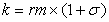(19)

Risk free rate is 5 percent annually and market return is 12 percent annually. From equation 19, we are able to obtain a discount factor value equal to 1.1440.

Project intrinsic value (IV) represents the static present vale estimated in the first four years. Terminal value (TV) symbolizes the subsequent four years where the free cash flow stabilizes on the fourth period estimated level (Eq. 20).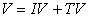(20)

Assuming a constant market share during the project lifespan, discounted cash flow values are expressed on Table 1.

Table 1. Project present value without considering strategic flexibility and assuming stability

Total present value goes up to \$144,755.11. This number includes an IV of \$156,979.63 and a TV of -\$12,224.53 (\$21,747.53 for period t = 4 discounted to initial period). Operative fixed costs present value goes up to \$69,186.14. Out of these numbers, we could state that it is more beneficial to license the project and do not make any additional investments in order to stay in business. The weakness of a static cash flow discount analysis is that risk is summed up and incorporated into the discount rate. We do not consider neither possible scenarios with associated probabilities nor strategic flexibility to continue or license the technology. We assume an exponential behavior over time and a normal metric that explains risk, where we do not include neither higher moments nor the abandonment of the assumption of normal statistical behavior.

3.3. Normal and with the Edgeworth Transformation Real Options

The project will be valued applying a binomial model in real options. We will transform the equation to sensitize higher moments (asymmetry and kurtosis), and we will analyze its impact its value. Since there is not a liquid market that we could use in order to calculate our project risk, we apply a MAD approach as a substitute of a similar portfolio. The estimated present value for the periods (\$156,979.63) represents the intrinsic value at which the project will be negotiable . Terminal value is not incorporated in the analysis since we assume strategic flexibility: expansion and investment or selling the license will depend on the final scenario at period 4.

Higher moments’ values with normal stochastic process are: asymmetry E = 0 and kurtosis K = 3. Comparatively through the example, we assign arbitrarily an asymmetry (E) of -0.23 and a kurtosis (K) of 3.5. As we will explain, higher moments will be modified and sensitize to analyze potential values presented by options. Applying equation 1 and assigning a value of x0=1, we are able to present the number of intermediate nodes and positions (j) occupied by the random variable. The number of final nodes is 5 (n+1).

We calculate the results with and without higher moments incorporating asymmetry (E= -0.23) and kurtosis (K = 3.5) (Eq. 4). On the first case, transformation is neutral (W(x) equal to 1). Therefore, function f(x) = b(x). On the second case, function f(x) adjusts values including the third and fourth stochastic moment.

Assigning a success probability (q) of 0.5, and projecting four periods of time, right section of Table 2 presents the probability related to each node using the binomial density function b(x). In order to integrate higher moments in the binomial distribution, we need to calculate the Edgeworth transformation (left section of Table 2). A new function comes out of the product of the binomial function b(x) and the expansion W(x). Consequently, f(x) = b(x).W(x).

Table 2. Function f(x): Product between the binomial function b(x) and the transformation W(x)

Function f(x) must be adjusted by the weighted factor in order to scale probabilities on each node, and they summed must be equal to 1 (Table 3).

Applying equations 5 and 6, we obtain necessary parameters for standardization and correction of biases included in the mean and variance. On the one hand, mean without stochastic higher moments is equal to 0. Although, while incorporating E=0.23 and K=3.5, mean on period 1 is -0.0023; on period 2, it is -0.0859; on period 3, it is -0.0978; and lastly, on period 4, it is 0.20304. On the other hand, variance is 1 when we do incorporate neither asymmetry nor kurtosis. Although, while incorporating E=0.23 and K=3.5, variance is 0.99999 on period 1; on period 2, it is 0.093424; on period 3, it is 1.00596; and lastly, for period 4, it is 1.41546.

Using equation 7, we standardize random variables (xj), and we are able to incorporate asymmetry and kurtosis applying the expansion W(x).

Table 4. Standardized variables: functions b(x) and f(x) (eq. 7)

Asset’s stochastic process’ projection (investment project value) implies estimating the growth rate (μ) to estimate potential values for each node. Growth rate, a previous step to estimating probable values, is obtained using equation 12.

Table 6. Stochastic process for project value: E=-0.23, K=3.5 (eq. 8)

Projected value for each node comes out of equation 8. Table 5 presents results assuming a normal distribution, and in Table 6, we expose an adjusted lattice with asymmetry and kurtosis.

Next, we will value strategic option contained in the project. In period 4, we present the dilemma of making incremental investments to remain in the market or selling the license. The first alternative is similar to a call option which exercised price (X) is given by actual incremental investment costs (VA(CI)). As it was previously indicated, this value goes up to \$69,186.14. If the economic benefit function is under the license transference price L (\$170,000), we exercise the put option. Option’s terminal value is stated as;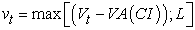(21)

In order to get the valuation, it is necessary to calculate the implied probabilities’ values. Firstly, we must apply equation 14 to estimate Qjt. In order to economize space, we just expose results with asymmetry and kurtosis on Table 7. Qjt results for the normal case are equal for every node of the same period (1 on period 0, 0.5 on period 1, 0.25 on period 2, 0.125 on period 3, and 0.0625 on period 4).

Subsequently, we must calculate certain equivalent coefficients (p, 1-p), applying equations 15 and 16. As in the previous case, we will estimate them without considering higher moments (obtaining a constant probability of 0.5), and incorporating them (Table 8).

While assuming normality, coefficients remain constant. As we incorporate higher moments through transformation, these vary on each node .

Table 8. p, 1-p for distribution E=0.23, K=3.5 (eq. 15 and 16)

We apply equation 21 in t=4 to estimate the option value at expiration. Then, we apply recursive procedure indicated in equation 18.

Table 9 and Table 10 expose the integral process of valuation. EV column presents option’s terminal value in period 4 (obtained on each node applying equation 21), multiplied by its probability Q(E). The sum of its product EVxQ(E) for each node comes up with \$196,913.03 and \$205,788.71, respectively without and with stochastic higher moments. Project’s value with options at initial moment is obtained applying equation 18. In order to do that, we use the previously mentioned risk free rate (5 percent) and estimated coefficients. Real option value (ROV) is the difference between project actual value with options Vt and project value without options V. As we state, the cash flow discount method undervalues the project since its value without flexibility was estimated in \$144,755.11 (Table 1). Once we apply real option theory and assuming normality, its total value is \$161,219 (Table 9) and its strategic flexibility’s value goes up to \$16,463.65. If we assume the existence of asymmetry and kurtosis, Table 10 presents a project’s value of \$168,486, and the real options actual value is equal to \$23,730.44.

Table 10. Option valuation: E=0.23, K=3.5 (eq. 18 and 21)

3.4. Scenarios Analysis

In Table 9 and Table 10, we expose probability distribution on t=4 related to different scenarios. On this case, if we do not verifies normal behavior Q(N), favorable probabilities Q(E) toward the put option exercising (selling the license) decrease turning more probable the call option exercising (investment).

Table 11. Real option value for different higher moments

On Table 11 and Illustration 1, we present the results derived from sensitizing higher moments. In order to do that, we describe variation intervals of 0.2 for asymmetry and 0.5 for kurtosis.

Figure 1. Asymmetry and kurtosis behavior as a value function

While sensitizing values, we could observe a real options value reduction mainly related to a decrease on the fourth stochastic moment. This is a consequence of lower volatility because of a steeper curve (lower kurtosis). A value reduction is seen from pair (E=0.8; K=4.5) to (E=0.4; K=2). From a superior positive asymmetry than 0.4, value starts growing. Therefore, third moment effect goes beyond the lower kurtosis (E=0.6; K=1.5). It is important to highlight how project value is affected by stochastic higher moments’ behavior which is frequently not considered by assuming a normal behavior related to the underlying asset.

4. Conclusion

Young investment projects based on new technologies’ development include contractual flexibilities that impact over their intrinsic value, and they require applying subjective probabilities. Project’s and strategic flexibility value is captured by real option method. Through this field of study, the binomial model is emphasized by its elasticity and simplicity to explain the complex phenomena of assigning value to strategic flexibility contained in investment projects. However, since it assumes a normal behavior of the stochastic process related to risk generating variables, a limitation is presented. This limitation occurs because if the random variable that commands uncertainty does not follow a normal behavior, higher moments are not captured and reflected in price. Consequently, the real asset could be either underpriced or overpriced. This paper developed a real option valuation model where the binomial probability distribution is adjusted through the Edgeworth transformation to capture higher moments (asymmetry and kurtosis). They allow us to explain project value and its strategic flexibility.

Throughout the paper, we analyzed a technology-based startup with a mixed participation (public educative sector and private risk capital) with options of expanding the investment (favorable scenarios) and selling the license (unfavorable scenarios). While selecting this kind of project, we noted that it is born completely from innovation, its main value is knowledge (intangible asset), there is neither history nor market prices nor comparable firms. Therefore, we could not apply the traditional cash flow discount approach, assume normal behavior of random variables, and value real options applying binomial method considering two stochastic moments. For these types of assets, we propose a model that:

It uses the MAD to estimate volatility related to the theoretical project’s value. This was calculated through the cash flow discount approach.

It corrects the binomial function b(x) incorporating Edgeworth transformation W(x) obtaining a new function f(x). This incorporates higher moments.

It projects the underlying asset value adjusting the first and second moments by the random variable corresponding to function f(x).

It calculates implied probabilities’ values and certain equivalent coefficient for each node. As we incorporate stochastic higher moments, last nodes are variable and used to apply the classical recursive method employed in valuing real options.

Sensitizes possible value pertained to the third and fourth stochastic moment in order to calculate all potential results.

Thus, we are able to balance out the binomial approach’s simplicity with the flexibility to assume, project, and sensitize probability distributions with the presence of asymmetry and kurtosis. Moreover, we are able to implement a complementary valuation proposal to classic methods. In particular, these proposals are innovative entrepreneurships, new markets lacking of historical values, observations, or random variable frequencies without market prices related to comparable assets and with high intangible value sustained on knowledge.

Statement of Competing Interests

The authors have no competing interests.

List of Abbreviations

TBF: Technology-Based Firms

References

  Bank, Matthias, and Katrin Wibmer (2011). Start-up firm valuation: A real-options approach. Austria: Working paper University of Innsbruck.In article  Bernardo, Antonio E., and Bhagwan Chowdhry (2002). “Resources, real options, and corporate strategy,” Journal of Financial Economics, 63, 211-234.In article CrossRef  Brandao, Luiz E., James S. Dyer, and Warren J. Hahn (2005). “Using Binomial Decision Trees to Solve Real Options Valuations Problems,” Journal of Decision Analysis, 2, 69-88.In article CrossRef  Copeland, Thomas E., and Vladimir Antikarov (2001). Real Options. New York: Texere LLC.In article  Fracica Naranjo, Germán, Patricia Vaca Vaca, and María P. Sepúlveda Calderón (2011). ‘El empresario en el start up,’ paper presented at the Memorias XXI Congreso Latinoamericano sobre Espíritu Empresarial. Cali, Colombia, March.In article  Landro, Alberto (2010). Acerca de la Probabilidad: La interpretación del concepto de azar y la definición de probabilidad. Buenos Aires: Centro de Investigaciones en Econometría Facultad de Ciencias Económicas UBA.In article  Lin, Barry J., and Anthony F. Herbst (2003). “Valuation of a startup business with pending patent using real options.” Accessed on Feb. 3, 2012.In article  Mun, Johnathan (2004). Real Options Analysis: Tools and Techniques for Valuing Strategic Investment and Decisions. New York: Wiley.In article  Pratt, Shannon P., and Roger Grabowski (2008). Cost Of Capital: Applications and Examples. New Jersey: John Wiley & Sons.In article  Rubinstein, Mark (1994). “Implied Binomial Trees,” Journal of Finance, 49 (3), 771-818.In article CrossRef  Rubinstein, Mark (1998). Edgeworth Binomial Trees. California: UC Berkeley.In article  Smit, Han T. J., and Lenos Trigeorgis (2004). Strategic Investment: Real Options and Games. New Jersey: Princeton University Press.In article  Smith, James E. (2005). “Alternative Approach for Solving Real Options Problems,” Decision Analysis, 2 (2), 89-102.In article CrossRef  Trigeorgis, Lenos (1997). Real Options: Managerial Flexibility and Strategy in Resource Allocations. Cambridge: MIT Press.In article  Wang, Ann, and William Halal (2010).”Comparison of Real Asset Valuation Models: A Literature Review,” International Journal of Business and Management, 5 (5), 14-24.In article  Wilmott, Paul (2009). Frequently Asked Questions in Quantitative Finance. United Kingdom: John Wiley & Sons.In article

Notes

1It means abandoning the objective probability definition, based on observations and event frequencies, and going over beliefs or agent’s judgment.

2With asymmetry E=0 and kurtosis K=3, transformation gets canceled, and the function goes back to the normal binomial state.

3Since there is not another company that replicates Project cash flow, deviation must be estimated using alternative methods like simulation. In options, it means to use MAD approach .

4Once we combine a call and a put option,our benefits of the strategy aims to cover the investment from extreme volatility. If the underlying asset price goes over investments (interpreted as the exercised price), we exercise the call option improving our device. If the price goes below our fixed assets investments to make the expansion possible, we exercise the put option and, therefore, we sell the product’s license.